# Pumps 5

Five pumps pumped in 3 hours 1800 hl of water. How many hectoliters of water pumped four equally powerful pumps in 6 hours?

x =  2880 hl

### Step-by-step explanation:Did you find an error or inaccuracy? Feel free to write us. Thank you!Tips to related online calculators
Need help to calculate sum, simplify or multiply fractions? Try our fraction calculator.
Tip: Our volume units converter will help you with the conversion of volume units.

## Related math problems and questions:

• PumpsFive pumps pumped 1,800 hl of water for 3 hours. How many hectoliters of water pump same two powerful pumps for six hours?
• PumpsAfter the floods, four equally powerful pumps exhausted water from the flooded cellar in 6 hours. How many hours would take a drained out with three equally powerful pumps?
• Pumps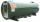6 pump fills the tank for 3 and a half days. How long will fill the tank 7 equally powerful pumps?
• Water tankIn an empty fire tank, 2150 hl of water jetted in 5 hours. How many hectoliters of water was jetted every hour? How many hectoliters of water was in the tank after three hours?
• Pumps 3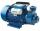Two pumps of the same power fill the garden pool for 10 hours. How many of these pumps would have to use if we want to shorten the filling of the pool to four hours?
• 5 pumps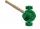Five pump to pump 3 hours 1600 hl of water. How long pumped same amount of water 4 pumps?
• HectolitersHow many hectoliters of water fits into cuboid tank with dimensions of a = 3.5 m b = 2.5 m c = 1.4 m?
• StudyFor 7 hours of study, she scored a 75, so if she made 93, how many hours would she need to study?
• Pump680 liters of water were pumped in 8 minutes. How many liters was spent in 56 minutes?
• Hours 3How many hours are in 3 2/3 days?
• A family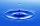A family of four consumes 220 liters of water a day. How many hectoliters of water are consumed by 68 tenants per day?
• Divide moneyDivide 1200 USD at a ratio of 1:2:3:4:5:6:9:10
• BuildingAt the building, we divided 240 boards into two piles in a 5: 3 ratio. How many were fewer boards in the lower pile?
• 5 2/55 2/5 hours a week  mathematics,  3 3/4 hours a week   Natural sciences, 4 3/8 hours a week  Technology . how many hours does  he spend on social sciences if he spend 17 1/2 hours a week for the four subject?
• Cyclist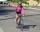Cyclist drives 5 km in half an hour, how long will drive in 3 hours?
• Change the numbers in the ratio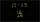Change the numbers 29, 38, and 43 in a 3: 4 ratio.
• Three-quarters of its volume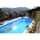The pool has a block shape with a length of 8m, a width of 5.3m and a depth of 1.5m. How many hl of water is in it if it is filled to three-quarters of its volume?